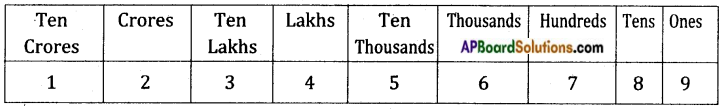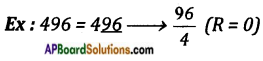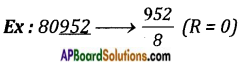Students can go through AP Board 8th Class Maths Notes Chapter 15 Playing with Numbers to understand and remember the concepts easily.

## AP State Board Syllabus 8th Class Maths Notes Chapter 15 Playing with Numbers

→ Place value of the digits:
12, 34, 56, 789→ Expanded form of the number:
7843 = 7 × 1000 + 8 × 100 + 4 × 10 + 3 × 1
= 7 × 103 + 8 × 102 + 4 × 101 + 3 × 100

→ Prime numbers: Numbers which are having the factors 1 and itself are called primes.
Ex: 2, 3, 5, 7, 11, 13, …. etc.→ Divisibility Rules:
(i) Divisibility by 10: If the units digit of a number is ‘0’ then it is divisible by 10.
Ex: 10, 50, 100, 150, 1000 etc.

(ii) Divisibility by 5: If the units digit of a number is either ‘0 ‘or ‘5’ then it is divisible by 5.
Ex: 15, 20, 50, 90 …. etc.

(iii) Divisibility by 2: If the units digit of a number is 0, 2, 4, 6, 8 then it is divisible by 2.
Ex: 0, 16, 32, 48, 22 …. etc.

(iv) Divisibility by 3: If the sum of the digits of a number is divisible by 3 then the number is also divisible by 3.
Ex: 126 → 1 + 2 + 6 → $$\frac{9}{3}$$ (R = 0) then 126 is divisible by 3.

(v) Divisibility by 6: If a number is divisible 2 and 3 then it is divisible by 6.

(vi) Divisibility by 4: If the last two digits of a number is divisible by 4, then the entire number is divisible by 4.∴ 496 is divisible by 4.(vii) Divisibility by 8: If the last 3 digits of a number is divisible by 8 then it is divisible by 8.∴ 80952 is divisible by 8.

(viii) Divisibility by 7: If a number is divisible by 7 (2a + 3b + c) must be divisible by 7.
Where a = digit at the hundreds place, b = digit at the tens place and c = digit at ones place.

(ix) Divisibility by ’11’: If the difference of the sum of digits in odd places and sum of digits in even places of a number is divisible by 11. Then the number is divisible by 11.
Ex: 1234321⇒ (1 + 3 + 3 + 1) – (2 + 4 + 2) = 8 – 8 = 0 → $$\frac{0}{11}$$ (R = 0)
∴ 1234321 is divisible by 11.